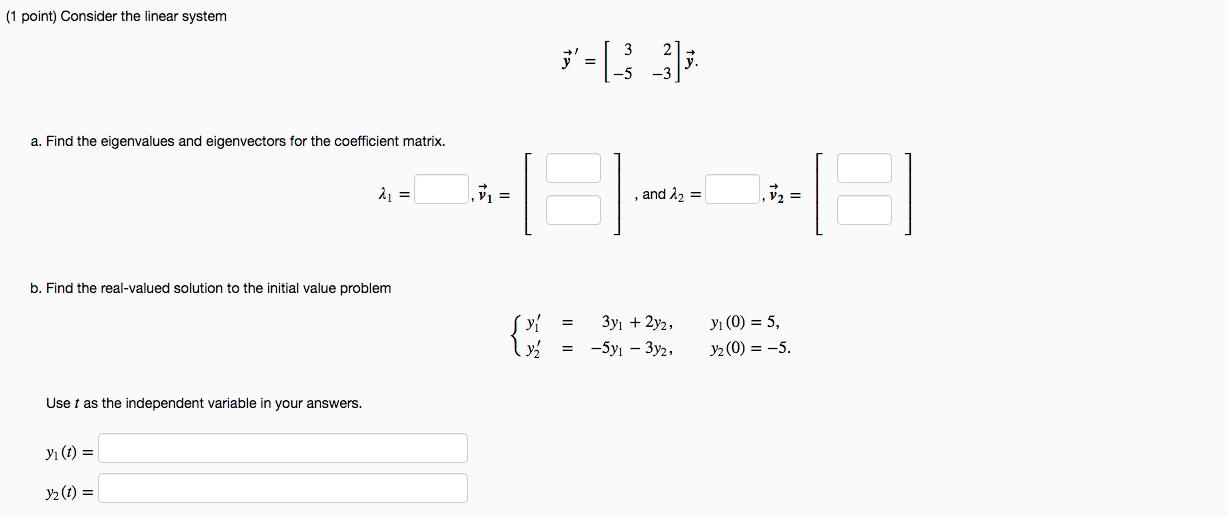I AM STRUGGLING HEAVILY ON THIS PROBLEM PLEASE HELP ME WITH THE CORRECT ANSWERS AND NEAT WORK

(1 point) Consider the linear system 3-1_3__3]; a. Find the eigenvalues and eigenvectors for the coefficient matrix. Vi = and 12 = -- b. Find the real-valued solution to the initial value problem (y 3yı + 2y2, -5yı - 3y2, yı (0) = 5, y2O) = -5. را Use t as the independent variable in your answers. yı(t) = yz(t) =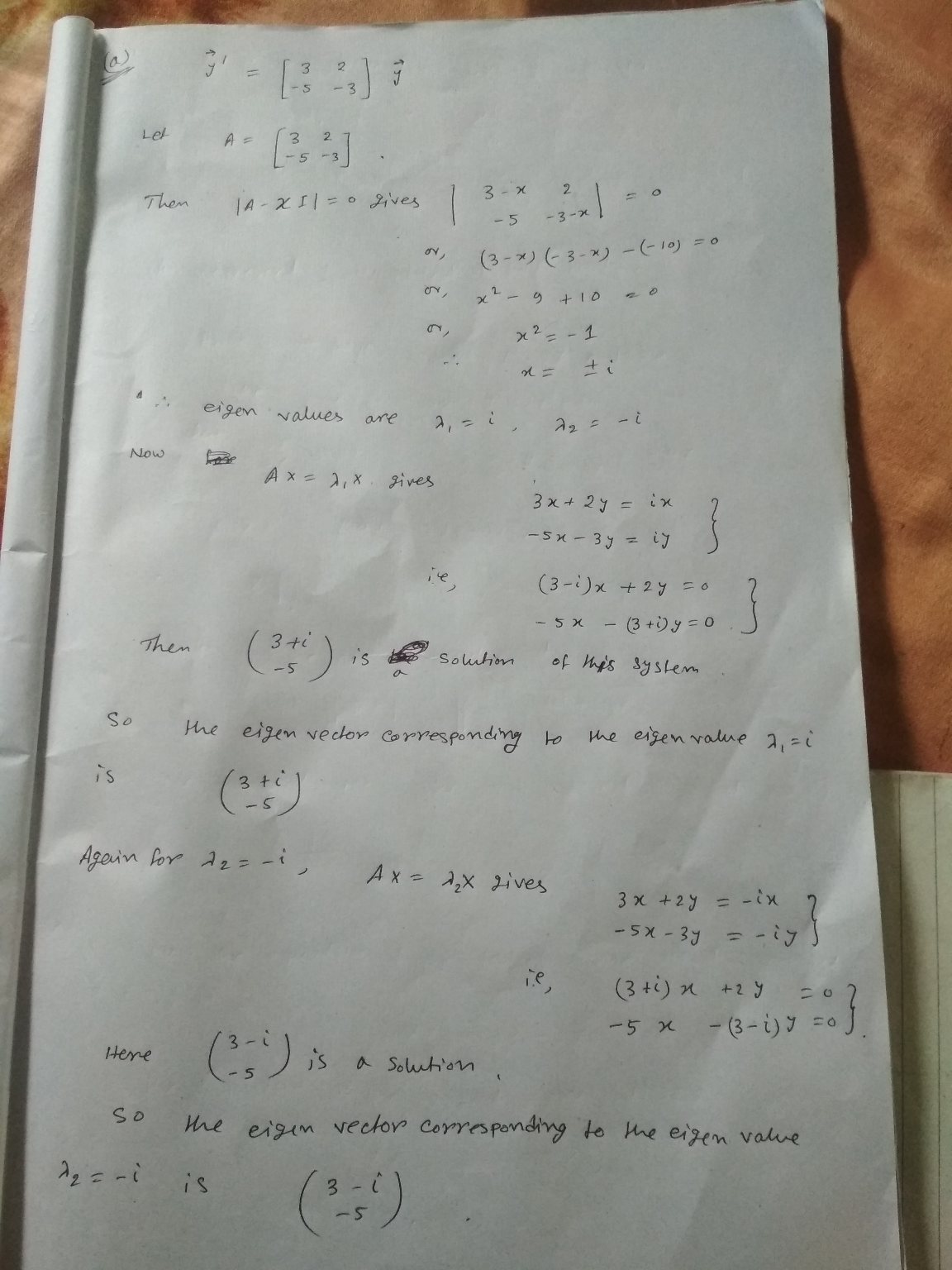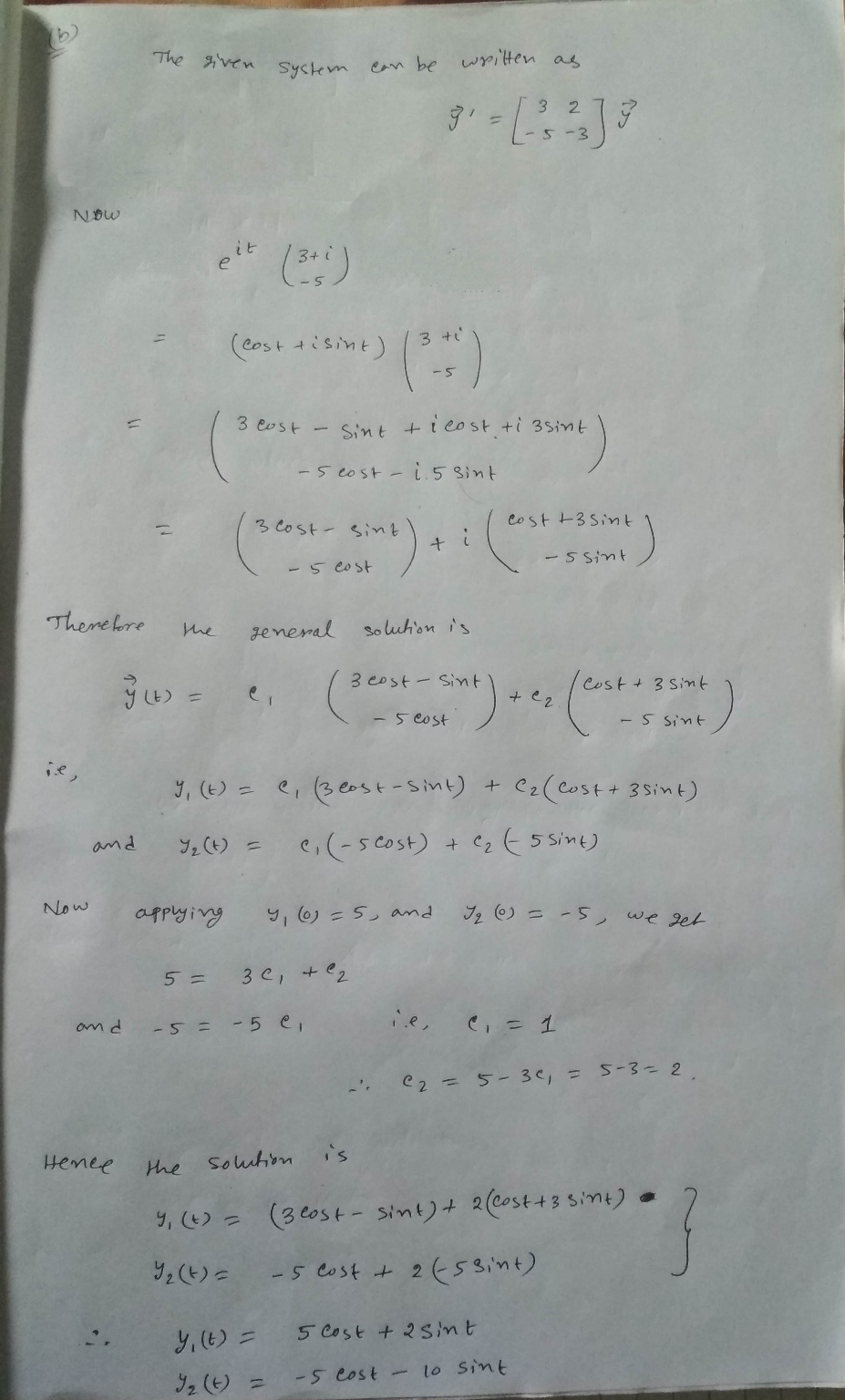Similar Homework Help Questions
• ### I AM REALLY STRUGGLING ON THIS PROBLEM PLEASE HELP ME CORRECT AND NEAT WORK IS MUCH...I AM REALLY STRUGGLING ON THIS PROBLEM PLEASE HELP ME CORRECT AND NEAT WORK IS MUCH APPRECIATED THANKS (1 point) Consider the linear system 3'=[} }); a. Find the eigenvalues and eigenvectors for the coefficient matrix. EL and 12 = b. Find the real-valued solution to the initial value problem y! 3yı + 2y2, -5yı - 3y2, yı(O) = 5, y2(0) = -5. Use t as the independent variable in your answers. yi(t) = y2(t) =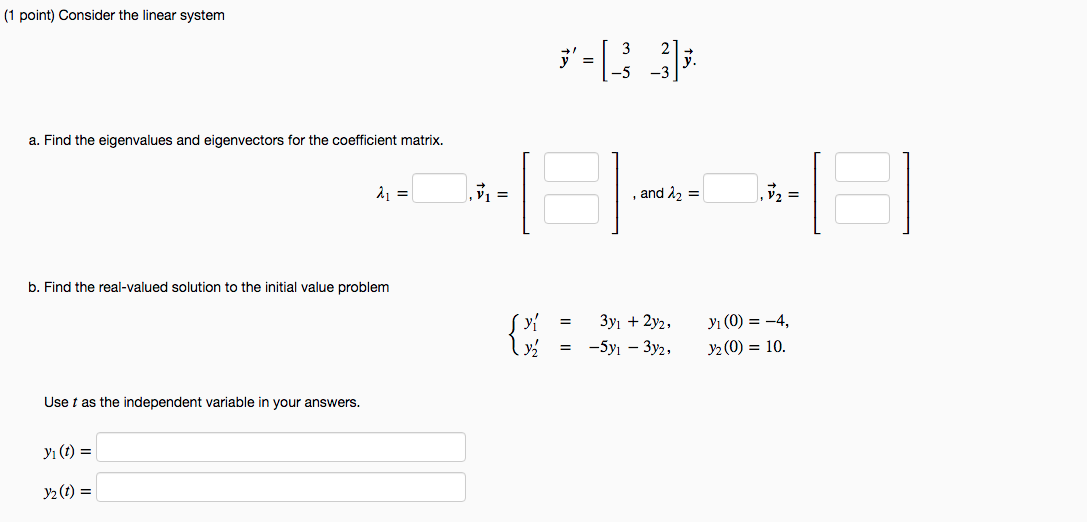PLEASE ANSWER AND FILL IN ALL ANSWER BOXES PLEASE ANSWER ALL QUESTIONS ASKED (1 point) Consider the linear system ;' = -5 -3): a. Find the eigenvalues and eigenvectors for the coefficient matrix. 21 = v= and 12 = V2= II b. Find the real-valued solution to the initial value problem 3yı + 2y2, y = -5yı - 3y2, yı(0) = -4, y2(0) = 10. Use t as the independent variable in your answers. yı(t) = y2(t) =

• ### STRUGGLING WITH THESE TWO SETS OF PROBLEMS ID APPRECIATE THE HELP AND ALL CORRECT ANSWERS PLEASE...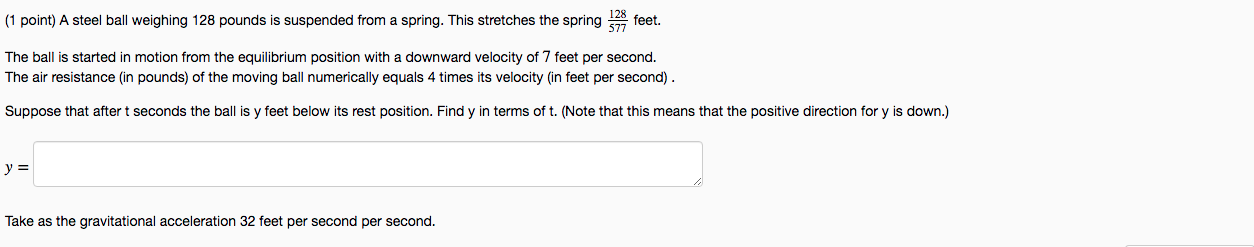STRUGGLING WITH THESE TWO SETS OF PROBLEMS ID APPRECIATE THE HELP AND ALL CORRECT ANSWERS PLEASE (1 point) A steel ball weighing 128 pounds is suspended from a spring. This stretches the spring 528 feet. The ball is started in motion from the equilibrium position with a downward velocity of 7 feet per second. The air resistance (in pounds) of the moving ball numerically equals 4 times its velocity (in feet per second). Suppose that after t seconds the ball...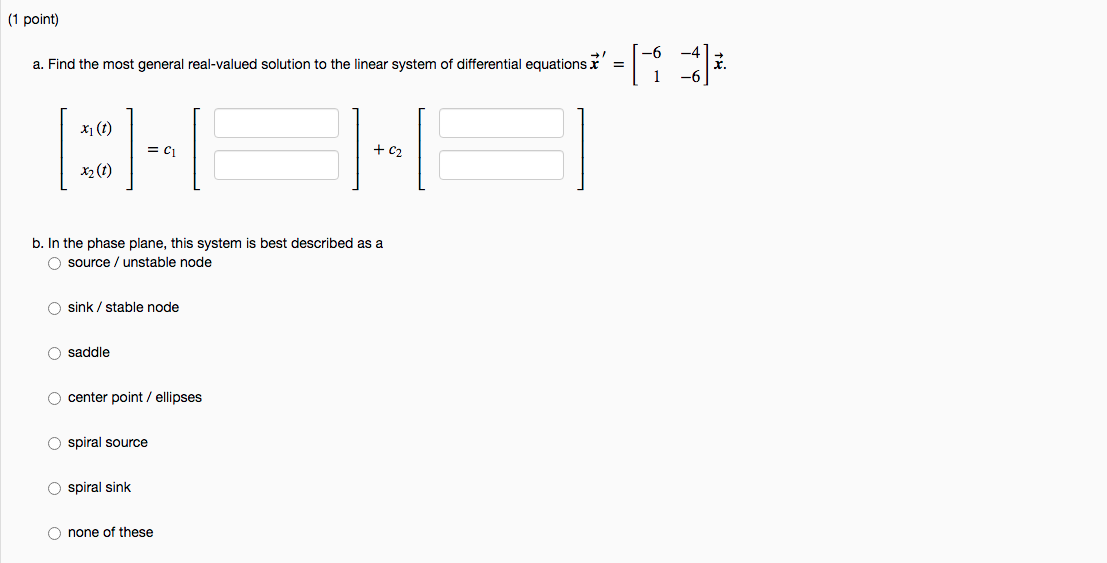I AM STRUGGLING HEAVILY ON THIS PROBLEM PLEASE HELP ME WITH THE CORRECT ANSWERS AND NEAT WORK PLEASE PLEASE AND THANK YOU (1 point) a. Find the most general real-valued solution to the linear system of differential equations t' = 1 -6 x1 (t) = C1 + C2 x2(t) b. In the phase plane, this system is best described as a O source / unstable node O sink / stable node O saddle O center point / ellipses Ospiral source...

• ### (1 point) Consider the linear system -3 -2 333 5 a. Find the eigenvalues and eigenvectors...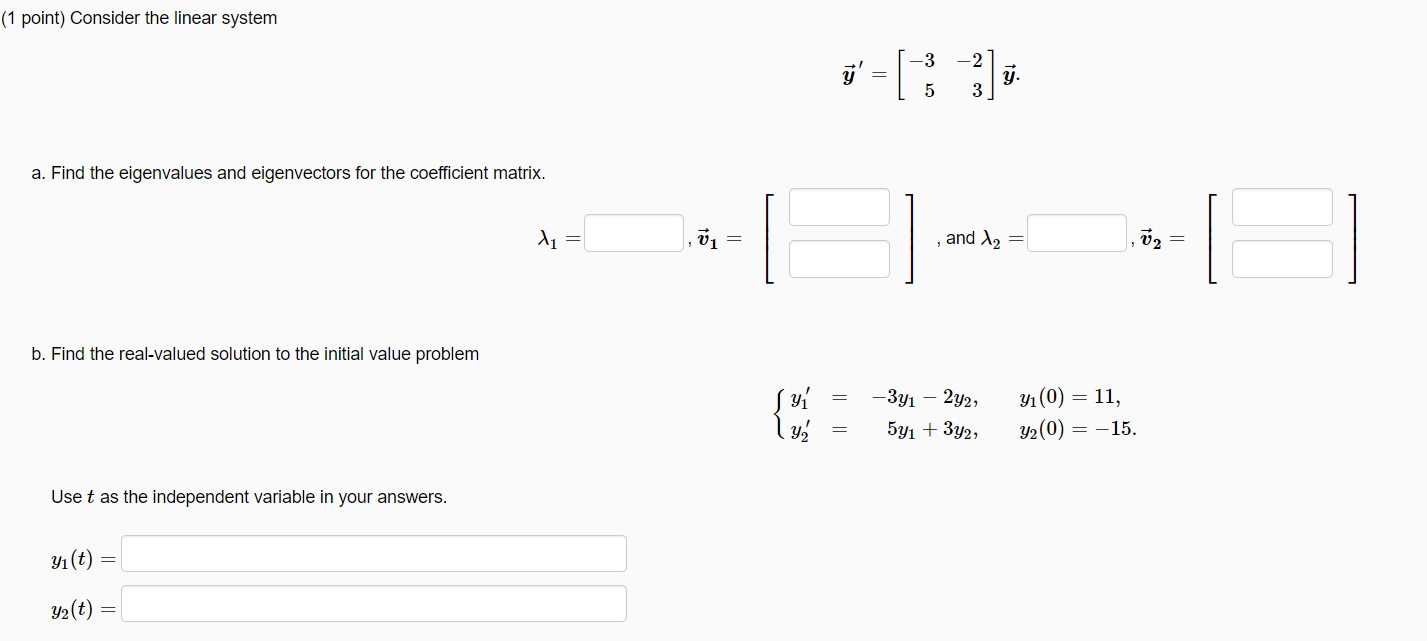(1 point) Consider the linear system -3 -2 333 5 a. Find the eigenvalues and eigenvectors for the coefficient matrix. di = and 12 02 b. Find the real-valued solution to the initial value problem syi ly -341 – 2y2, 5y1 + 3y2, yı(0) = 11, y2(0) = -15. Use t as the independent variable in your answers. yı(t) y2(t)

• ### I am not sure about the eigen vectors or the eigen values would like confirmation and...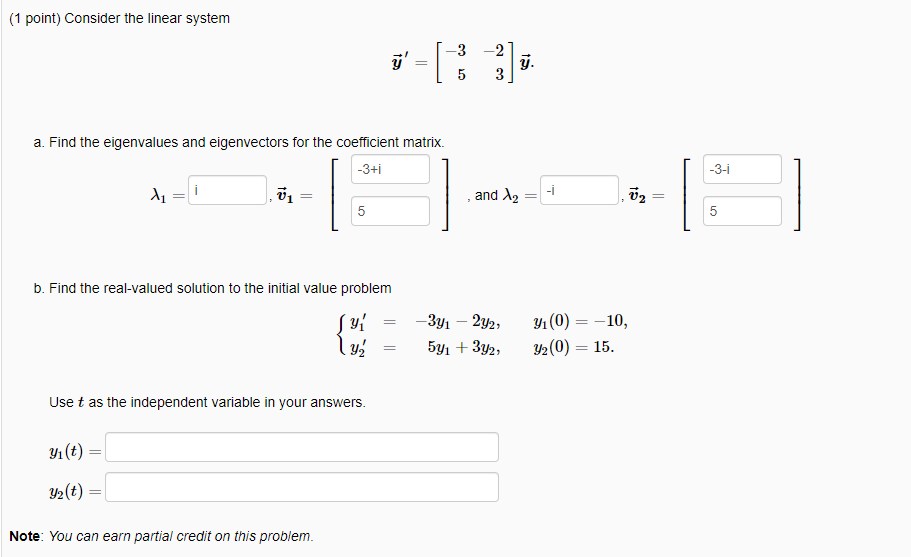I am not sure about the eigen vectors or the eigen values would like confirmation and the solutions for part B as well, Thank you. (1 point) Consider the linear system =  -3 -2 5 3 y. -3-1 a. Find the eigenvalues and eigenvectors for the coefficient matrix. -3+1 di vi 5 -i and 12 13 5 b. Find the real-valued solution to the initial value problem โปร์ -3y1 - 2y2 5y1 + 3y2, yı(0) = -10, y2(0) =...

• ### (1 point) Consider the linear system 3 2 ' = y. -5 -3 a. Find the...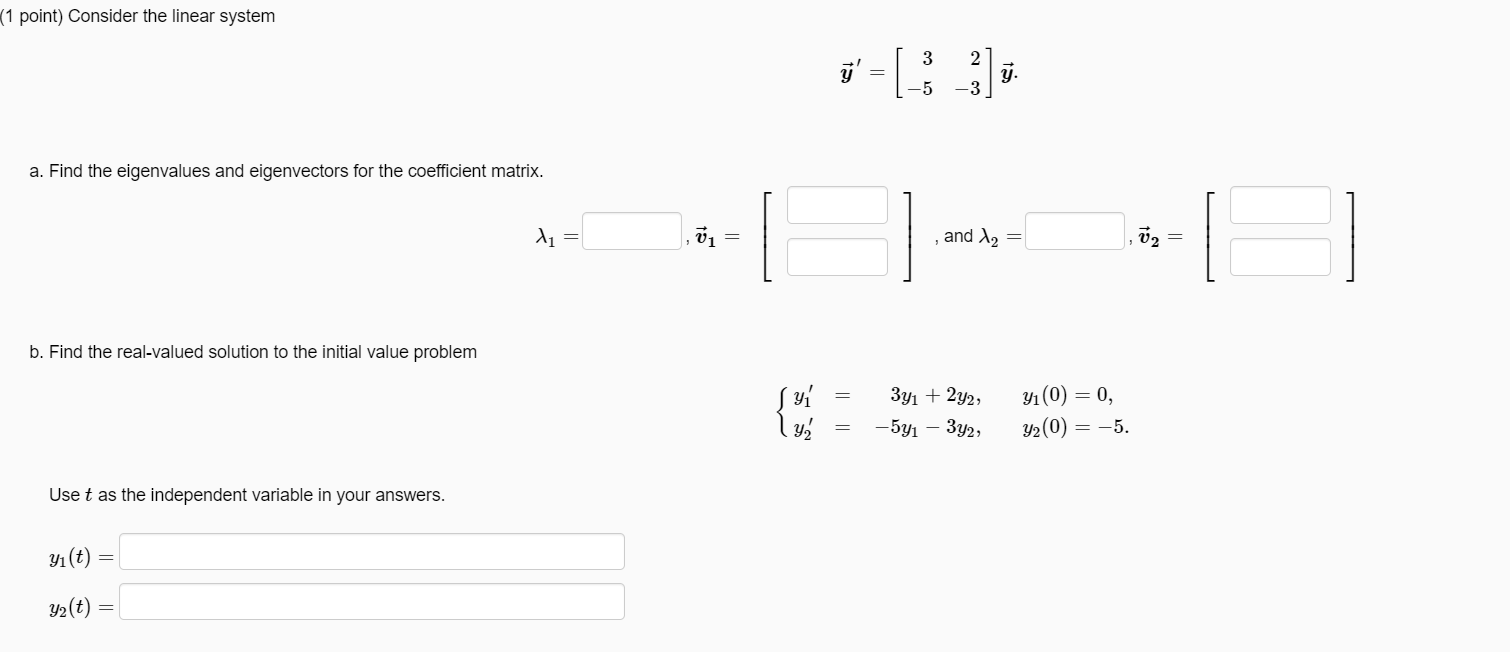(1 point) Consider the linear system 3 2 ' = y. -5 -3 a. Find the eigenvalues and eigenvectors for the coefficient matrix. 2 = 15 and 2 V2 b. Find the real-valued solution to the initial value problem Syi ly 3y1 + 2y2, -541 – 3y2, yı(0) = 0, y2(0) = -5. Use t as the independent variable in your answers. yı(t) y2(t)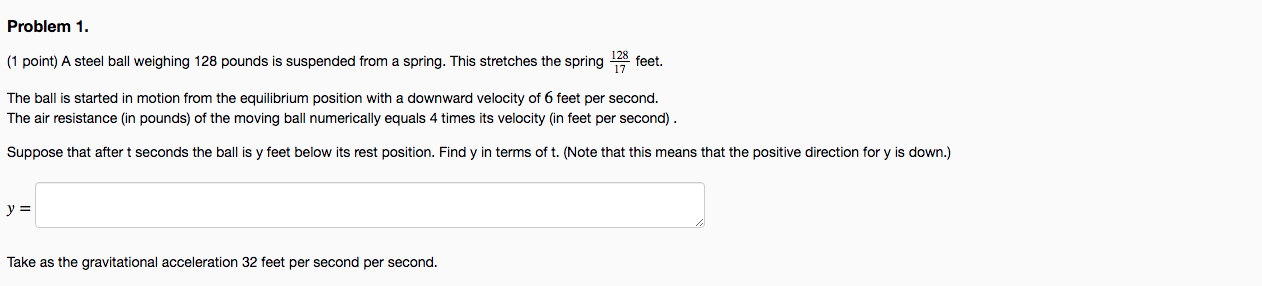I AM STRUGGLING HEAVILY ON THIS PROBLEM PLEASE HELP ME WITH THE CORRECT ANSWER AND NEAT WORK PLEASE PLEASE AND THANK YOU Problem 1. (1 point) A steel ball weighing 128 pounds is suspended from a spring. This stretches the spring 178 feet. The ball is started in motion from the equilibrium position with a downward velocity of 6 feet per second. The air resistance (in pounds) of the moving ball numerically equals times its velocity (in feet per second)...

• ### Problem 5. (1 point) Consider the linear system a. Find the eigenvalues and eigenvectors for the...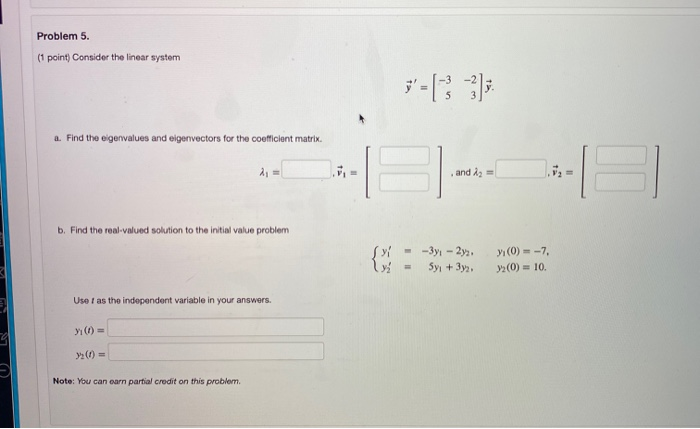Problem 5. (1 point) Consider the linear system a. Find the eigenvalues and eigenvectors for the coefficient matrix. and iz = b. Find the real-valued solution to the initial value problem - -3y - 2y2 Syı + 3y2 yı(0) = -7, (0) = 10 Use I as the independent variable in your answers. Y() = Note: You can earn partial credit on this problem. Problem 6. (1 point) Find the most general real-valued solution to the linear system of differential...

• ### I've figured out the first part but am struggling with the second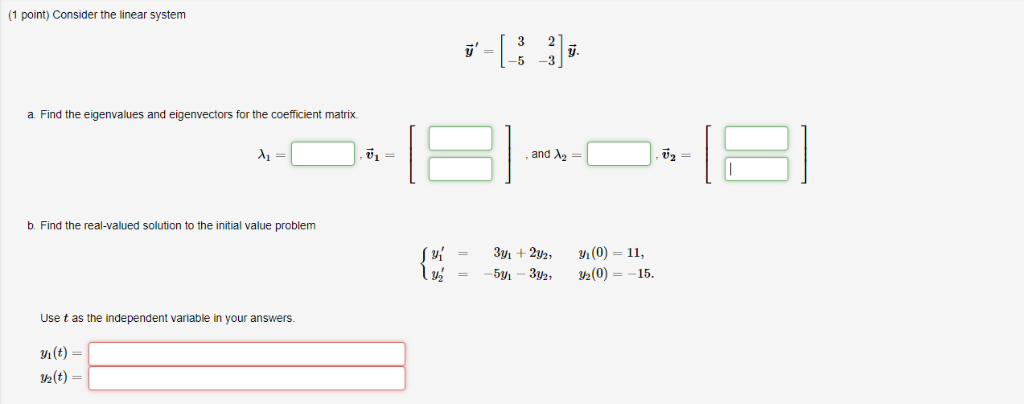I've figured out the first part but am struggling with the second (1 point) Consider the linear system -5 3 a. Find the eigenvalues and eigenvectors for the coefficient matrix. and λ2 = b. Find the real-valued solution to the initial value problem y( 334 + 2h, m(0) = 11, 2(0)15. = Use t as the independent variable in your answers. i(t) /2(t) 2 t) (1 point) Consider the linear system -5 3 a. Find the eigenvalues and eigenvectors for...

Free Homework App